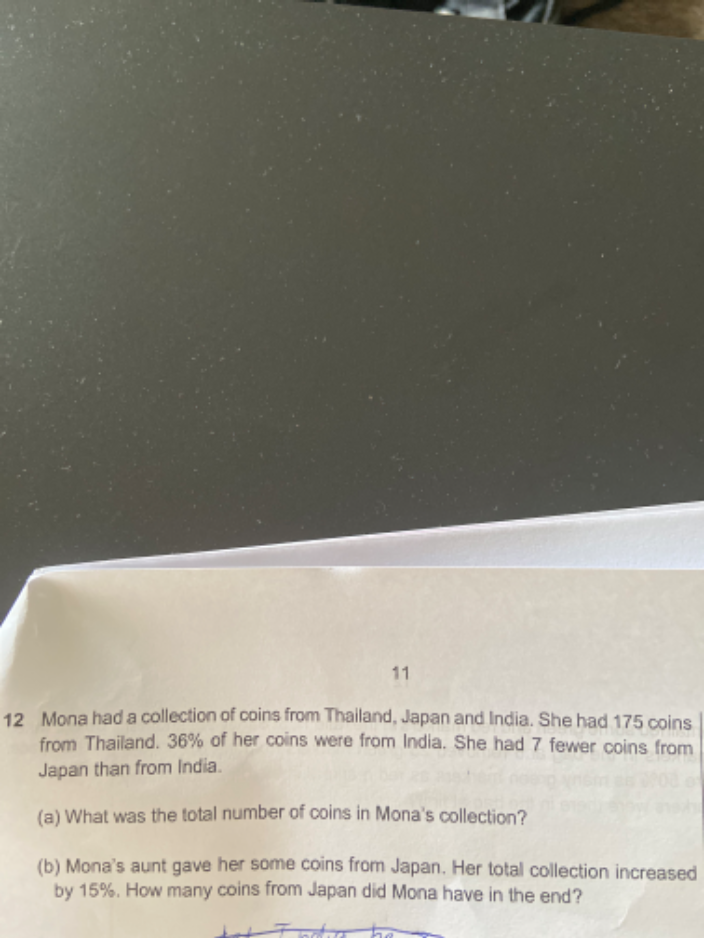# Questionthanks in advance to solve this question

(a)   Let u = total number of coins

T                  I                  J

[    175     ][  0.36u   ][   0.36u-7  ]

u – 0.36u – (0.36u-7) = 175

u – 0.36u – 0.36u +7 = 175

0.28u = 168

u =600

Hence total number of coins in Mona’s collection is 600

(b) 0.15 x 600 = 90

At first,  J coins = 0.36 x 600 – 7 = 216-7 = 209

Hence in the end Mona has 209+90 = 299 coins from Japan.

0 Replies 2 Likes ✔Accepted Answer